Next: Potential outside uniform spheroid Up: Newtonian gravity Previous: Axially symmetric mass distributions

# Potential due to uniform sphere

Let us calculate the gravitational potential generated by a sphere of uniform mass density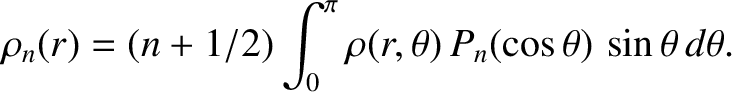, and radius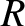, whose center coincides with the origin. Expressing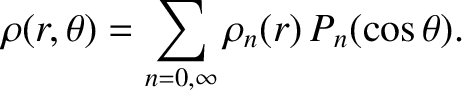in the form of Equation (3.47), we find that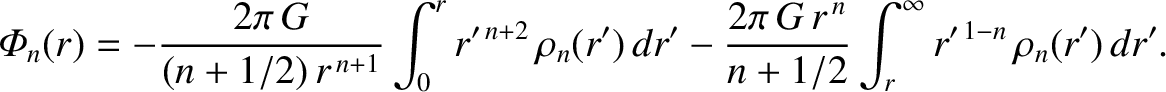(3.50)

with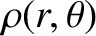for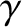. Thus, from Equation (3.49),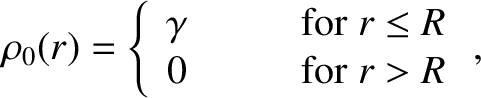(3.51)

for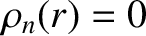, and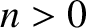(3.52)

for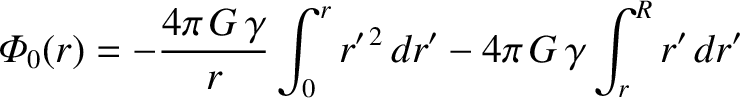, with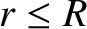for. Hence,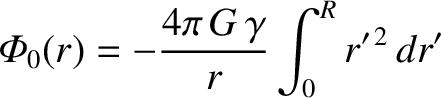(3.53)

for, and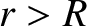(3.54)

for. Here,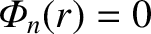is the total mass of the sphere.

According to Equation (3.54), the gravitational potential outside a uniform sphere of mass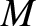is the same as that generated by a point masslocated at the sphere's center. It turns out that this is a general result for any finite spherically symmetric mass distribution. Indeed, from the preceding analysis, it is clear that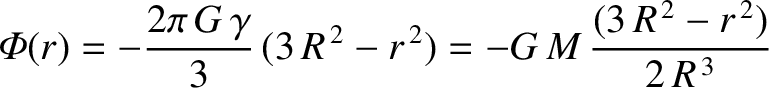and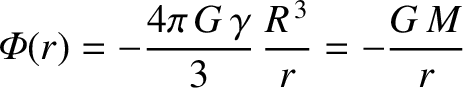for such a distribution. Suppose that the distribution extends out to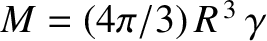. It immediately follows, from Equation (3.49), that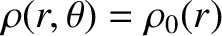(3.55)

for, whereis the total mass of the distribution.

Consider a point massthat lies a distance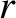from the center of a spherically symmetric mass distribution of mass(whereexceeds the outer radius of the distribution). Because the external gravitational potential generated by the distribution is the same as that of a point masslocated at its center, the force exerted on the massby the distribution is the same as that due to a point masslocated at the center of the distribution. In other words, the force is of magnitude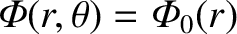, and is directed from the mass toward the center of the distribution. Assuming that the system is isolated, the resultant force that the mass exerts on the distribution is of magnitude, and has a line of action directed from the center of the distribution towards the mass. (See Exercise 3.) However, this is the same as the force that the masswould exert on a point masslocated at the center of the distribution. Because gravitational fields are superposable, we conclude that the resultant gravitational force acting on a spherically symmetric mass distribution of masssituated in the gravitational field generated by many point masses is the same as that which would act on a point masslocated at the center of the distribution.

The center of mass of a spherically symmetric mass distribution lies at the geometric center of the distribution. Moreover, the translational motion of the center of mass is analogous to that of a point particle, whose mass is equal to that of the whole distribution, moving under the action of the resultant external force. (See Section 2.6.) If the external force is due to a gravitational field then the resultant force is the same as that exerted by the field on a point particle, whose mass is that of the distribution, located at the center of mass. We thus conclude that Newton's laws of motion, in their primitive form, apply not only to point masses, but also to the translational motions of extended spherically symmetric mass distributions interacting via gravity (e.g., the Sun and the planets).Next: Potential outside uniform spheroid Up: Newtonian gravity Previous: Axially symmetric mass distributions
Richard Fitzpatrick 2016-03-31